Latest Banking jobs   »

# Quantitative Aptitude Quiz For IRDA AM 2023 -11th May

Directions (1-5): Bar graph given below gives information about raw material cost (in Rs. ‘000) of five different products, i.e. (A, B, C, D, and E) manufactured by a company and percentage for cost of production which was calculated on raw material cost of that product. (Cost price of each product for company = raw material cost of that product+ cost of production of that product).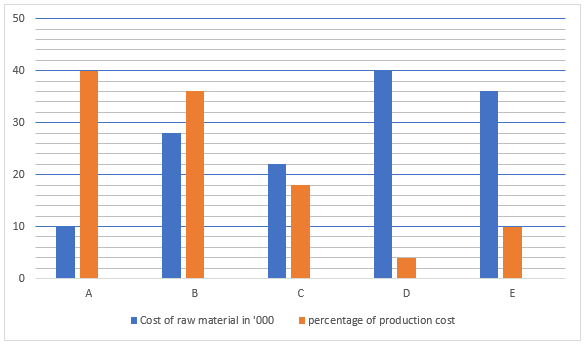Q1. If on selling product B company got profit of 16 ⅔% of production cost of that product. Find market price of the product if product was sold at market price.
(a) Rs.38080
(b) Rs.26532
(c) Rs.29480
(d) Rs.35784
(e) Rs.39760

Q2. Find ratio of cost price of product A to cost price of product E for the company.
(a) 5:18
(b) 1:4
(c) 35:99
(d) 15:38
(e) 19:45

Q3. Production cost consists of transportation cost and machining cost. If for product D transportation cost is 10% of its production cost, then find at what price company should sell it to get 25% profit if transportation cost is not considered by company in calculating cost price of this product?
(a) Rs.51800
(b) Rs.41000
(c) Rs.42518
(d) Rs.40400
(e) Rs.43428

Q4. Production cost of product B is approximately how much percent more than production cost of product C?
(a) 160%
(b) 155%
(c) 142%
(d) 157%
(e) 162%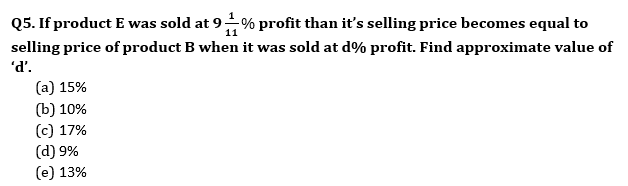Directions (6 –10): Line graph shows marked up percentage of four articles above cost price and table shows marked price of these four articles. Read the data carefully and answer the questions.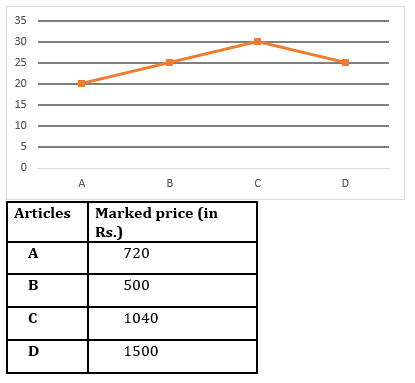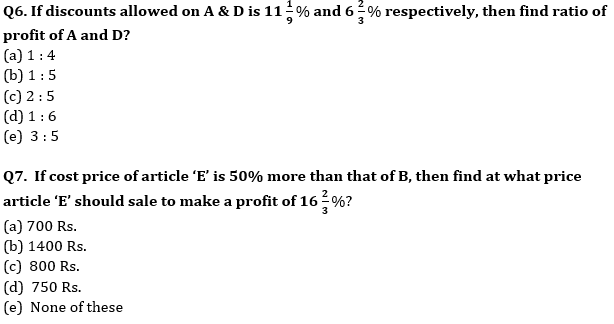Q8. If discount (in %) given on article B & D is in the ratio of 1 : 3 and ratio of profit on article B & loss on article D is same (in Rs) , then find discount allowed on article D?
(a) 18%
(b) 12%
(c) 30%
(d) 15 %
(e) 24%

Q9. Cost price of A is what percent less than average cost price of B, C & D ?
(a) 12%
(b) 10%
(c) 15%
(d) 25%
(e) 20%

Q10. Discount allowed on article C is 15% and selling price of article ‘X’ is Rs. 316 more than that of C. If profit made on ‘X’ is 20%, then find difference between cost price of D & X?
(a) 300 Rs.
(b) 600 Rs.
(c) 400 Rs.
(d) 200 Rs.
(e) 100 Rs.

Solutions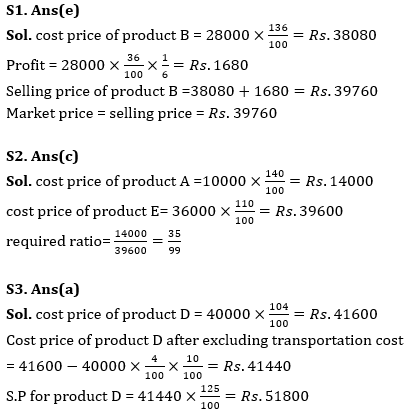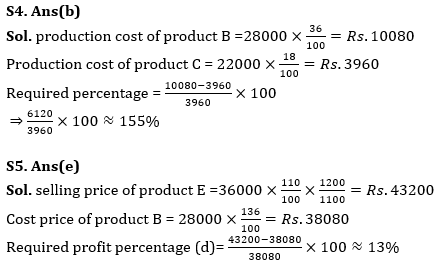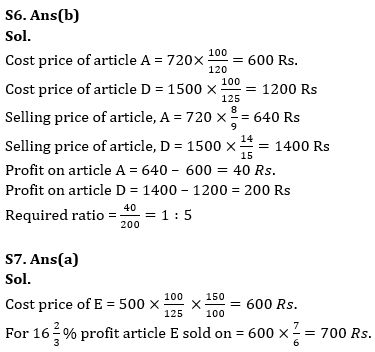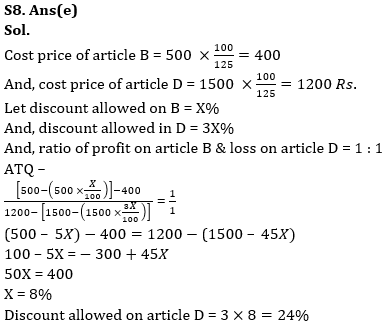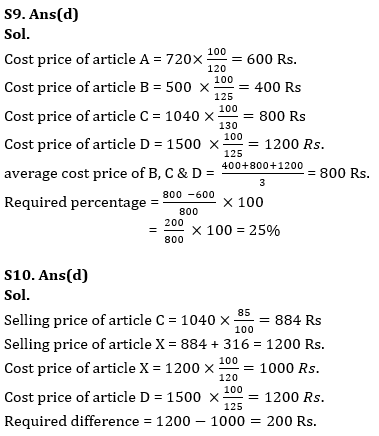## FAQs

### How many sections are there in the IRDAI exam?

There are 4 sections in the IRDAI assistant manager online preliminary exam. They are Reasoning, English Language, General awareness, Quantitative aptitude.

#### Congratulations!Union Budget 2023-24: Free PDF